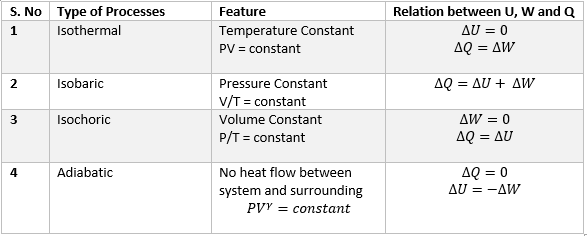# Thermodynamic Processes

## (a) Quasi static Processes

• In Quasi static process deviation of system from it's thermodynamic equilibrium is infinitesimally small.
• All the states through which system passed during a quasi static process may be regarded as equilibrium states.
• Consider a system in which gas is contained in a cylinder fitted with a movable piston then if the piston is pushed in a infinitely slow rate, the system will be in quiescent all the time and the process can be considered as quasi-static process.
• Vanishingly slowness of the process is an essential feature of quasi-static process.
• During quasi-static process system at every moment is infinitesimally near the state of thermodynamic equilibrium.
• Quasi static process is an idealized concept and its conditions can never be rigorously satisfied in practice.
• In practice, processes that are sufficiently slow and do not involve accelerated motion of the piston, large temperature gradient, etc. are reasonably approximation to an ideal quasi-static process.
• The processes define below quasi-static processes only, except when stated otherwise.

## (b) Isothermal Process

• In isothermal process temperature of the system remains constant throughout the process.
• For an isothermal process equation connecting P, V and T gives.
$PV = constant$
i.e., pressure of given mass of gas varies inversely with its volume this is nothing but the Boyle's law.
• In isothermal process there is no change in temperature, since internal energy for an ideal gas depends only on temperature hence in isothermal process there is no change in internal energy.
Thus,
$\Delta U=0$
therefore From First law of Thermodynamics
$\Delta Q =\Delta W$
• Thus during isothermal process
Heat added (or subtracted) from the system = work done by (or on) the system
• PV diagram for the Isothermal process is shown below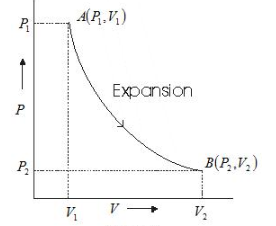• TV diagram for the Isothermal process is shown below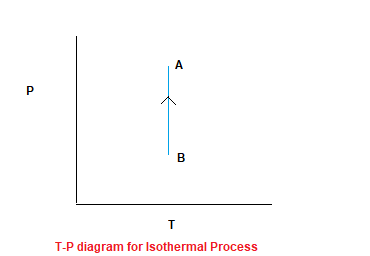• Process in which no heat enters or leaves a system is called an adiabatic process
• For every adiabatic process $\Delta Q=0$
• Prevention of heat flow can be accomplished by surrounding system with a thick layer of heat insulating material like cork, asbestos etc.
• Flow of heat requires finite time so if a process is perfomed very quickly then process will be practically adiabatic.
• On applying first law to adiabatic process we get
$\Delta U=U_2 - U_1= - \Delta W$
• In adiabatic process change in internal energy of a system is equal in magnitude to the work by the system.
• If work is done on the system contracts i.e. $\Delta W$ is negative then.
$\Delta U = \Delta W$
and internal energy of system increases by an amount equal to the work done on it and temperature of system increases.
• If work is done by the system i.e., $\Delta W$ is negative
$\Delta U = -\Delta W$
here internal energy of systems decreases resulting a drop in temperature.
• For adiabatic process of ideal Gas, the relation between Pressure and Volume is given by
$PV^{\gamma}= Constant$
Where $\gamma = \frac {C_p}{C_v}$
• if an ideal gas undergoes a change in its state adiabatically from $(P_1, V_1)$ to $(P_2,V_2)$, we have
$P_1V_1^{\gamma}= P_2V_2^{\gamma}$
• PV diagram for the adiabatic Process is shown as below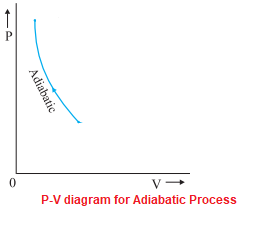• We can see the PV diagram of Isothermal Process and Adiabatic Process is similar. The graph of Isothermal is more tilted.
Here is the P-V graph of an ideal gas for two adiabatic processes connected two isotherms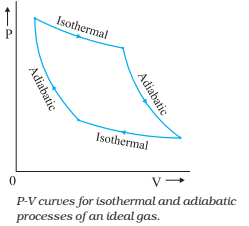## (d) Isochoric process

• In an Isochoric process volume of the system remain uncharged throughout i.e. $\Delta V = O$.
• When volume does not change no work is done , $\Delta W = 0$ and therefore from first law of Thermodynamics
$U_2 - U_2 = \Delta U =\Delta Q$
• All the heat given to the system has been used to increase the internal energy of the system.
• For an Isochoric process equation connecting P, V and T gives.
$\frac {P}{T}= constant$
i.e Pressure increases as the temperature increased
• PV diagram for the Isochoric Process is shown as below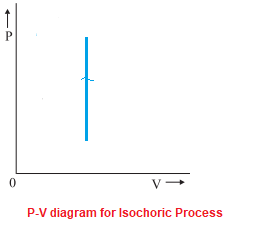• T-P diagram for the Isochoric Process is shown as below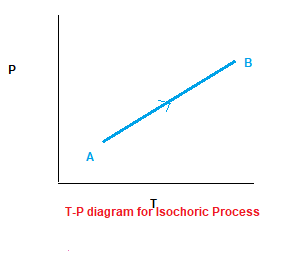It is straight line having some slope

## (e) Isobaric Process

• A process taking place at constant pressure is called isobaric process.
• From equation (3) we see that work done in isobaric process is
$W = P(V_2 - V_1) =nR (T_2-T_1)$
where pressure is kept constant.
• Here in this process the amount of heat given to the system is partly used in increasing temperature and partly used in doing work.
i,e $\Delta Q = \Delta U + \Delta W$
• For an isobaric process equation connecting P, V and T gives.
$\frac {V}{T}= constant$
i.e Volume increases as the temperature increases
• P-V diagram for the Isobaric Process is shown as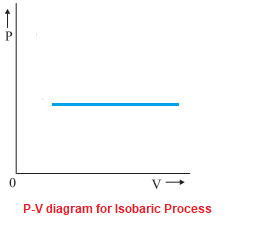• V-T diagram for the Isobaric Process is shown as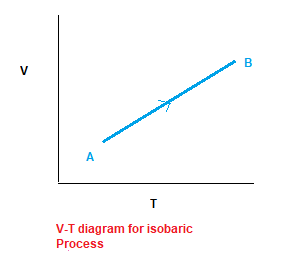## (e) Cyclic Process

• In a cyclic process, the system returns to its initial state
• Now since the system returns to it initial state,change in internal energy is zero
$\Delta U=0$
• Here in this process total heat absorbed is equal total work done by the system.
i,e $\Delta Q = \Delta W$
• P-V diagram for the Cyclic Process is shown as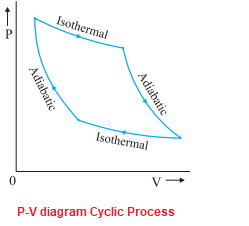In Summary , we can represent Thermodynamics Process as